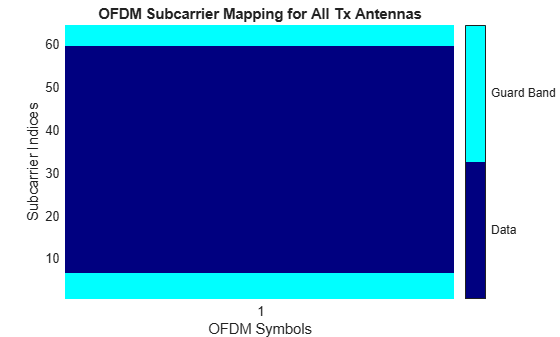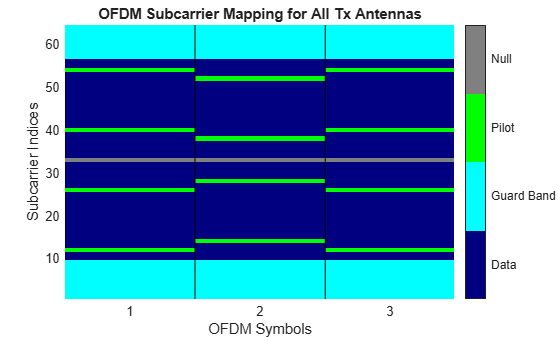# comm.OFDMDemodulator

Demodulate using OFDM method

## Description

The `comm.OFDMDemodulator` System object™ demodulates a time domain signal by using the orthogonal frequency division multiplexing (OFDM) method. For more information, see OFDM Demodulation. The output is a baseband representation of the input to the `comm.OFDMModulator` companion object.

To demodulate an OFDM signal:

1. Create the `comm.OFDMDemodulator` object and set its properties.

2. Call the object with arguments, as if it were a function.

## Creation

### Syntax

``ofdmDemod = comm.OFDMDemodulator``
``ofdmDemod = comm.OFDMDemodulator(Name=Value)``
``ofdmDemod = comm.OFDMDemodulator(odfmMod)``

### Description

example

````ofdmDemod = comm.OFDMDemodulator` creates an OFDM demodulator System object that demodulates an input signal by using the orthogonal frequency division demodulation method.```

example

````ofdmDemod = comm.OFDMDemodulator(Name=Value)` specifies Properties using one or more name-value arguments. For example, `comm.OFDMDemodulator(FFTLength=128)` configures the object for a total of 128 subcarriers.```

example

````ofdmDemod = comm.OFDMDemodulator(odfmMod)` sets the OFDM demodulator object properties based on the specified OFDM modulator object.```

## Properties

expand all

Unless otherwise indicated, properties are nontunable, which means you cannot change their values after calling the object. Objects lock when you call them, and the `release` function unlocks them.

If a property is tunable, you can change its value at any time.

Number of fast Fourier transform (FFT) points, specified as a positive, integer scalar. The length of the FFT must be greater than or equal to 8 and is equivalent to the number of subcarriers.

Number of subcarriers allocated to the left and right guard bands, specified as a 2-by-1 integer vector. The number of left and right guard-band subcarriers, [NleftG; NrightG], must fall within [0,NFFT / 2 ⌋ − 1], where NFFT is the total number of subcarriers in the OFDM signal specified by `FFTLength`. For more information, see Subcarrier Allocation, Guard Bands, and Guard Intervals.

Option to remove the null DC subcarrier, specified as a numeric or logical `0` (`false`) or `1` (`true`). The null DC subcarrier is at the center of the frequency band and has the index value:

• (NFFT / 2) + 1 when NFFT is even.

• (NFFT + 1) / 2 when NFFT is odd.

NFFT is the total number of subcarriers in the OFDM signal specified by `FFTLength`.

Option to output pilot subcarriers, specified as a numeric or logical `0` (`false`) or `1` (`true`). When you set this property to:

• `false` — Pilot information may be present but remains embedded in the output data.

• `true` — The object separates the pilot subcarriers, specified by `PilotCarrierIndices`, from the output data and outputs the demodulated pilot signal to the `pilot` output variable.

Indices of the pilot subcarrier locations, specified as a column vector, matrix, or 3D array with integer-element values in the range

`$\left[{N}_{\text{leftG}}+1,\text{\hspace{0.17em}}{N}_{\text{FFT}}/2\right]\cup \left[{N}_{\text{FFT}}/2+2,\text{\hspace{0.17em}}{N}_{\text{FFT}}-{N}_{\text{rightG}}\right],$`

where NFFT is the total number of subcarriers specified by `FFTLength`, and NleftG and NrightG are the left and right guard bands specified by `NumGuardBandCarriers`.

You can assign the NPilot pilot carrier indices to the same or different NSym subcarriers for each symbol, and across NT transmit antennas.

• When the pilot indices are the same for every symbol and transmit antenna, the property has dimensions of NPilot-by-1.

• When the pilot indices vary across symbols, the property has dimensions of NPilot-by-NSym.

• If the received signal assigned one symbol across multiple transmit antennas, the property has dimensions of NPilot-by-1-by-NT.

• If the indices vary across the number of symbols and transmit antennas, the property has dimensions of NPilot-by-NSym-by-NT.

Tip

To minimize interference between transmissions across more than one transmit antenna, the pilot indices per symbol must be mutually distinct across the antennas.

#### Dependencies

This property applies when you set `PilotOutputPort` to `1`.

Length of the cyclic prefix for each OFDM symbol, specified as a positive, integer scalar or row vector containing `NumSymbols` elements. When you specify the cyclic prefix length as a:

• Scalar — The cyclic prefix length is the same for all symbols through all antennas.

• Row vector — The cyclic prefix length may vary across symbols but does not vary across antennas.

Oversampling factor, specified as a positive scalar. The oversampling factor must satisfy these constraints:

Tip

If you set the oversampling factor to an irrational number, specify the fractional value. For example, with an FFT length of `12` and an oversampling factor of `4/3`, their product is the integer `16`. However, rounding `4/3` to `1.333` when setting the oversampling factor results in a noninteger product of `15.9960`, which results in a code error.

Data Types: `double`

Number of OFDM symbols in the time-frequency grid, specified as a positive, integer scalar.

Number of receive antennas to receive the OFDM modulated signal, specified as a positive, integer scalar less than or equal to `64`.

## Usage

### Syntax

``Y = ofdmDemod(X)``
``[Y,pilot] = ofdmDemod(X)``

### Description

example

````Y = ofdmDemod(X)` demodulates the input time domain signal using the OFDM method and returns the OFDM-demodulated baseband signal.```

example

````[Y,pilot] = ofdmDemod(X)` separates the `pilot` signal on the subcarriers specified by the `PilotCarrierIndices` property value. To enable this syntax, set the `PilotOutputPort` property to true.```

### Input Arguments

expand all

OFDM-modulated baseband signal, specified as a (NCPTotal + NFFT × NSym)-by-NR matrix.

You can determine the dimensions by using the `info` object function.

### Output Arguments

expand all

Output baseband signal, returned as a matrix or NData-by-NSym-by-NR array. The output reduces to a matrix when NR is `1`.

Data Types: `double`
Complex Number Support: Yes

Pilot signal, returned as an NPilot-by-NSym-by-NR array.

#### Dependencies

To return this output, set the `PilotOutputPort` property to `true`.

Data Types: `double`
Complex Number Support: Yes

## Object Functions

To use an object function, specify the System object as the first input argument. For example, to release system resources of a System object named `obj`, use this syntax:

`release(obj)`

expand all

 `info` Provide dimensioning information for OFDM demodulator `showResourceMapping` Show subcarrier mapping of OFDM symbols created by OFDM modulator or demodulator System object
 `step` Run System object algorithm `release` Release resources and allow changes to System object property values and input characteristics `reset` Reset internal states of System object

## Examples

collapse all

Create an OFDM demodulator System object™ with default properties. Modify some of the properties. Inspect the object configuration by using the `info` object function.

`ofdmDemod = comm.OFDMDemodulator`
```ofdmDemod = comm.OFDMDemodulator with properties: FFTLength: 64 NumGuardBandCarriers: [2x1 double] RemoveDCCarrier: false PilotOutputPort: false CyclicPrefixLength: 16 OversamplingFactor: 1 NumSymbols: 1 NumReceiveAntennas: 1 ```
`info(ofdmDemod)`
```ans = struct with fields: InputSize: [80 1] DataOutputSize: [53 1] ```

Modify the number of subcarriers, symbols, and receive antennas. Also enable the pilot output.

```ofdmDemod.FFTLength = 128; ofdmDemod.PilotOutputPort = 1; ofdmDemod.NumSymbols = 2; ofdmDemod.NumReceiveAntennas = 2;```

Verify that the number of subcarriers and the number of symbols changed. Reinspect the input and output signal dimensions by using the `info` object function. Notice the addition of the pilot output dimensions to the information structure. becasue the number of receive antennas is greater than 1, the data and pilot output dimensions are 3D arrays rather than matrices.

`ofdmDemod`
```ofdmDemod = comm.OFDMDemodulator with properties: FFTLength: 128 NumGuardBandCarriers: [2x1 double] RemoveDCCarrier: false PilotOutputPort: true PilotCarrierIndices: [4x1 double] CyclicPrefixLength: 16 OversamplingFactor: 1 NumSymbols: 2 NumReceiveAntennas: 2 ```
`info(ofdmDemod)`
```ans = struct with fields: InputSize: [288 2] DataOutputSize: [113 2 2] PilotOutputSize: [4 2 2] ```

Creating the demodulator by using the configured modulator ensures a matched modulator and demodulator pair.

Create an OFDM modulator specifying four transmit antennas.

`ofdmMod = comm.OFDMModulator(NumTransmitAntennas=4);`

Use the OFDM modulator to create the OFDM demodulator.

`ofdmDemod = comm.OFDMDemodulator(ofdmMod);`

Display the properties of the OFDM modulator and demodulator, verifying that the applicable properties match. The number of transmit antennas is independent of the number of receive antennas.

`ofdmMod`
```ofdmMod = comm.OFDMModulator with properties: FFTLength: 64 NumGuardBandCarriers: [2x1 double] InsertDCNull: false PilotInputPort: false CyclicPrefixLength: 16 Windowing: false OversamplingFactor: 1 NumSymbols: 1 NumTransmitAntennas: 4 ```
`ofdmDemod`
```ofdmDemod = comm.OFDMDemodulator with properties: FFTLength: 64 NumGuardBandCarriers: [2x1 double] RemoveDCCarrier: false PilotOutputPort: false CyclicPrefixLength: 16 OversamplingFactor: 1 NumSymbols: 1 NumReceiveAntennas: 1 ```

The `showResourceMapping` object function shows the time-frequency resource mapping for each transmit antenna.

Create an OFDM demodulator.

`demod = comm.OFDMDemodulator;`

Display the OFDM subcarrier mapping by using the `showResourceMapping` object function.

`showResourceMapping(demod)`Remove the DC subcarrier.

`demod.RemoveDCCarrier = true;`

Show the resource mapping after removing the DC subcarrier.

`showResourceMapping(demod)`Create an OFDM modulator with an inserted DC null, seven guard-band subcarriers, and two symbols that have different pilot indices for each symbol.

```ofdmMod = comm.OFDMModulator( ... NumGuardBandCarriers=[4; 3], ... PilotInputPort=true, ... PilotCarrierIndices=cat(2,[12; 26; 40; 54],[11; 27; 39; 55]), ... NumSymbols=2, ... InsertDCNull=true);```

Determine input data, pilot, and output data dimensions.

`modDim = info(ofdmMod)`
```modDim = struct with fields: DataInputSize: [52 2] PilotInputSize: [4 2] OutputSize: [160 1] ```

Generate random data symbols for the OFDM modulator. Determine the number of data symbols by using the structure variable, `modDim`.

```dataIn = complex( ... randn(modDim.DataInputSize),randn(modDim.DataInputSize));```

Create a pilot signal that has the correct dimensions.

```pilotIn = complex( ... rand(modDim.PilotInputSize),rand(modDim.PilotInputSize));```

Apply OFDM modulation to the data and pilot signals.

`modSig = ofdmMod(dataIn,pilotIn);`

Use the OFDM modulator object to create the corresponding OFDM demodulator.

`ofdmDemod = comm.OFDMDemodulator(ofdmMod);`

Demodulate the OFDM signal and output the data and pilot signals.

`[dataOut,pilotOut] = ofdmDemod(modSig);`

Verify that the input data and pilot symbols match the output data and pilot symbols.

```isSame = (max(abs([dataIn(:) - dataOut(:); ... pilotIn(:) - pilotOut(:)])) < 1e-10)```
```isSame = logical 1 ```

Filter an OFDM modulated signal with data and pilot inputs and outputs generated at four times the sample rate through a single-input single-output (SISO) channel. Demodulate the channel-filtered signal and compare to the original data.

Create an OFDM demodulator object that has three symbols and different pilot subcarrier indices and cyclic prefix lengths for each symbol.

```ofdmDemod = comm.OFDMDemodulator( ... NumGuardBandCarriers=[9;8], ... RemoveDCCarrier=true, ... PilotOutputPort=true, ... PilotCarrierIndices=[[12;26;40;54],[14;28;38;52],[12;26;40;54]], ... CyclicPrefixLength=[16 32 16], ... OversamplingFactor=4, ... NumSymbols=3);```

Create an OFDM modulator System object from the OFDM demodulator object, `ofdmDemod`.

`ofdmMod = comm.OFDMModulator(ofdmDemod);`

Show the configured subcarrier resource mapping for data, pilot, guard band and null signals by using the `showResourceMapping` object function. Obtain the input and output dimension information by using the `info` object function.

`showResourceMapping(ofdmDemod);``modDim = info(ofdmMod);`

Create random data and pilot inputs and apply QAM modulation.

```M = 16; xd = randi([0 M-1],modDim.DataInputSize); dataIn = qammod(xd,M,UnitAveragePower=true); xp = randi([0 M-1],modDim.PilotInputSize); pilotIn = qammod(xp,M,UnitAveragePower=true);```

Apply OFDM modulation to the data and pilot QAM signals. Filter the signal through an AWGN channel. To recover the data and pilot symbols, apply OFDM demodulation and then QAM-demodulation.

```modOut = ofdmMod(dataIn,pilotIn); chanOut = awgn(modOut,20,"measured"); [dataOut, pilotOut] = ofdmDemod(chanOut); yd = qamdemod(dataOut,M,UnitAveragePower=true); yp = qamdemod(pilotOut,M,UnitAveragePower=true);```

Verify that the data and pilots are unchanged through this process.

`dataSame = isequal(xd,yd)`
```dataSame = logical 1 ```
`pilotSame = isequal(xp,yp)`
```pilotSame = logical 1 ```

## Algorithms

expand all

 Dahlman, E., S. Parkvall, and J. Skold. 4G LTE/LTE-Advanced for Mobile Broadband.London: Elsevier Ltd., 2011.

 Andrews, J. G., A. Ghosh, and R. Muhamed, Fundamentals of WiMAX, Upper Saddle River, NJ: Prentice Hall, 2007.

 IEEE Standard 802.16-2017. "Part 16: Air Interface for Broadband Wireless Access Systems." March 2018.